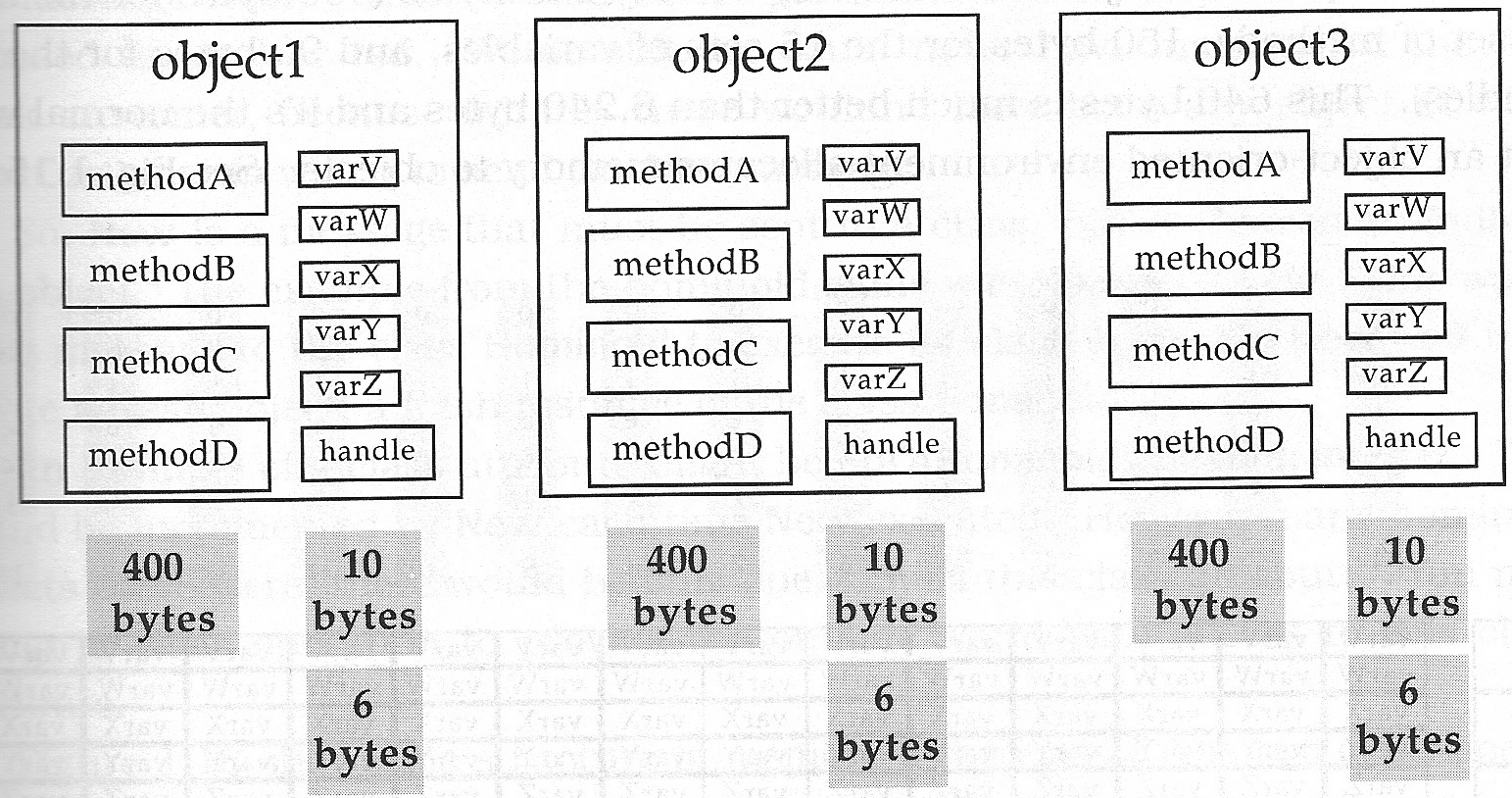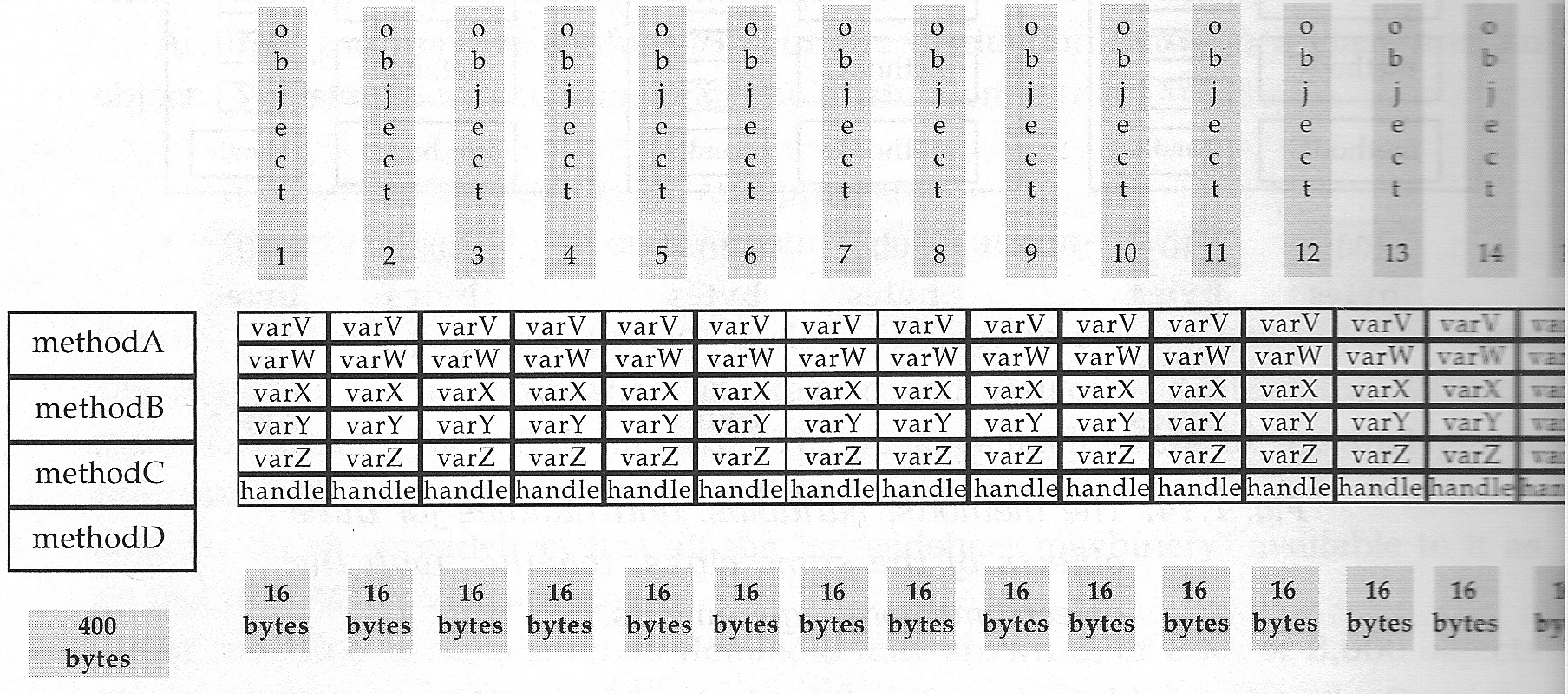## Chapter 7. Classes

Whenever we excute the "new" command on a class we instanciate an object that is structually identical to every other object created by the "new" statement on the same class.

Example: new Hominoid() creates an instance that is stucturally identical to every other new Hominoid().

Structurally identical means: same operations and variables

### Important

class

A class is the stencil from which objects are created (instantiated). Each object has the same structure and behavior as the class from which is is instantiated.

If object obj belongs to class C we say "obj is an instance of C."

There are two differences between two objects of the same class:

• Each object has a different handle

• Each object will probably have a different sate ( = values for its variables)

The difference between objects and classes:

• A class is what you design and program

• Objects are what you create (from a class) at run-time

Architecture: Class is the blueprint, object is the house.

A class may spawn 3, 300, 3000, a lot of objects. A class resembles a stencil: Once the shape of the stencil is cut, the same shape can be traced from it thousands of times. All of the tracings will be identical (but still different objects).

But why bother?

Look at the memory requirements: Lets assume that an object of a particular class has

• 5 variables that require 2 bytes each = 10 bytes

• 4 methods that require a total of 400 bytes

• a handle requiring 6 bytes 

This means a total of 416 bytes required for that object.

Now lets assume we have three objects of that class:

Figure 7.1. Three objects of the same class and their memory requirementsNow each one of them requires 416 bytes, making a total of 416 * 3 = 1248 bytes, right?

No! Because all of these objects are of the same class they are structurally identically, they may share the common code, which is the methods. (Each object has its own handle, and its own values for the variables, so they can not be shared)

So by sharing the actual code, which is the largest portion in most cases, we are able to save big!

Lets assume we now have 15 instances:

Figure 7.2. 15 objects sharing the same classWe need:

• 400 bytes for the class methods ( * 1)

• 16 bytes for handle + values ( * 15)

Making a total of 400 + 16 * 15 = 640 bytes!

Practice: Assume a class with the following memory requirements:

• methods: 500 bytes

• variables: 96 bytes

• handle: 4 bytes

How much memory is used for 1 instance? for 5 instances?

600 bytes / 1 and 1000 / 5

So far we have talked about so called "object instance operations" and "object instance variables".

## Class operations and class attributes

Object instance operations and object instance variables work with instances of classes.

What happens if we want something where we know we have only one of it? Do we need to create an object for every "helper" class and pass it around to every other object?

No, we can use class operations and class variables instead!

They work much like in procedural programming (there can be only one), but are still encapsulated in a "class".

Object instance and class operations / attributes can even be mixed in one class.

Class operations are used:

• When there is only one of a certain thing.

• When there is no state.

Example of only one: Bank in Monopoly (guess what we're doing in next lab :) ). Screen on the computer.

Example of no state: Utility functions, such a math functions, helper functions, etc. but also creation functions.

Class operation can only work with class attributes (they have no instance)!

Object instance operations can work with class attributes or object instance attributes.

You can call class operations without creating an instance first! So anyone can send a message to a class operation without having a handle.

Sometime the notion "static" is used for class attributes and operations. (MagicDraw uses the flag "is static" for class attributes and operatons, C++ and Java use the keyword "static")

Notation in UML: In UML class attributes and operations are underlined.

Figure 7.3. Grid for the Hominoid, implement completely static since there is only onePractice:

Assume a game of scrabble. The game has exactly one bag. The bag contains a bunch of letters (char) and provides an operation for retrieving a letter, and one to check if the bag is empty. Draw a UML diagram for the class "ScrabbleBag".

Figure 7.4. Solution for ScrabbleExample: Using static methods for creation of instances:

Figure 7.5. A color class with static methods for creationPractice: Draw a UML diagram of a "Location" class. It should provide attributes for x and y and a class operation for "get origin"

Figure 7.6. Example solution for Location classBook: Chapter 1.6, 3.6

 In reality a handle uses 4 bytes on most current (32 bit) systems, and 8 bytes on newer (64 bit) systems. How they came up with 6 bytes I do not know.